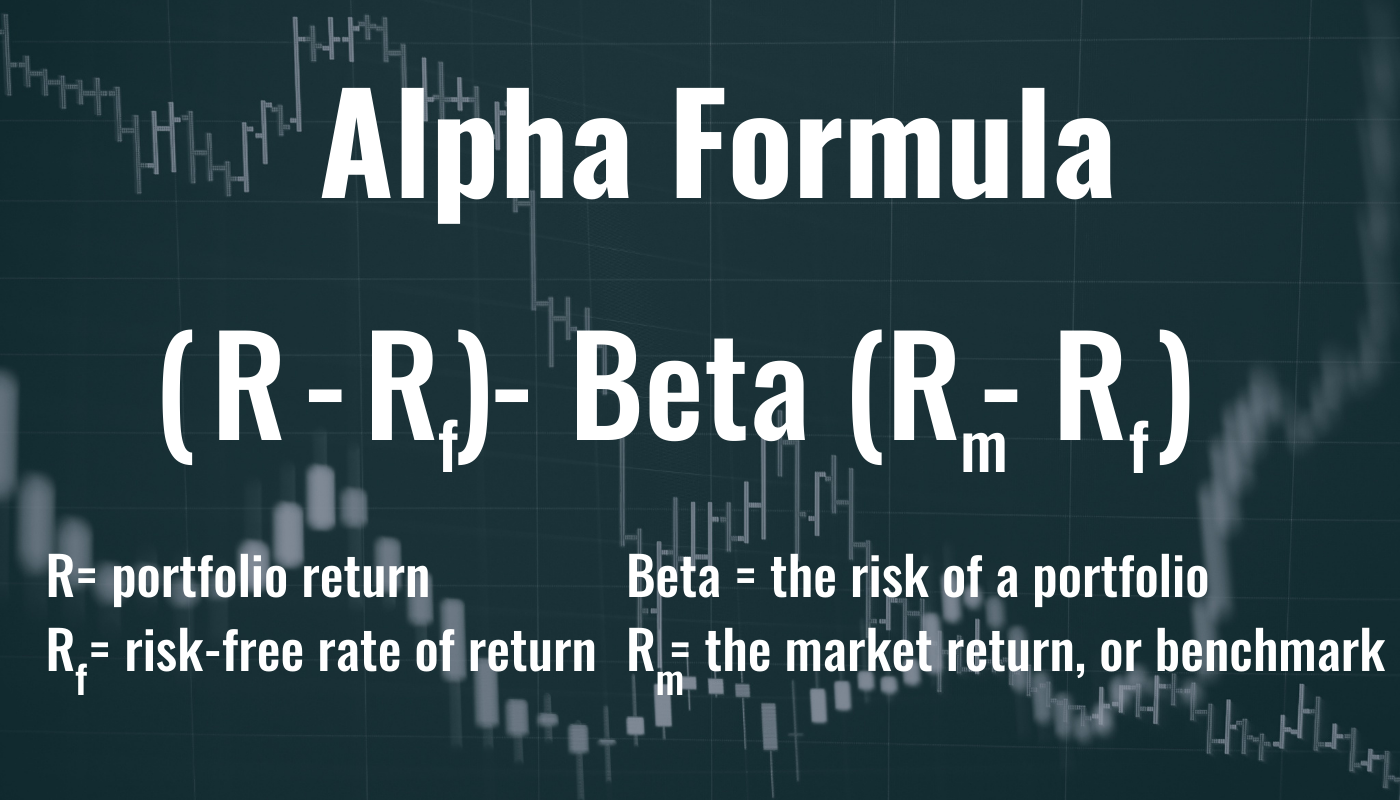# What Is Alpha in Finance? Definition, Formula & Examples

Alpha is a tool that shows investors how well their investments are doing relative to a benchmark index.A positive alpha demonstrates that a fund has performed better than its benchmark.tang90246 from Getty Images; Canva

## What Is Alpha?

The first letter in the Greek alphabet, alpha (α), has come to signify something of primary or significant importance. In finance, an investment with high alpha is one that has exceeded its benchmark in terms of returns.

Alpha is a risk ratio that measures how well a security, such as a mutual fund or even a stock, has performed relative to a market index, such as the S&P 500. Alpha is paired with beta (β), the second letter of the Greek alphabet, which measures an asset’s volatility.

In actively managed mutual funds and ETFs, alpha is viewed as an indicator of fund manager performance. A positive alpha demonstrates that the fund has performed better than its benchmark, while a negative alpha shows that the fund has underperformed. It’s also important to note that alpha is measured after management fees (e.g., expense ratios) are taken out.

The concept of alpha originated from the introduction of weighted index funds; alpha was created as a way to compare active investing with passive index investing.

## Example of Alpha

Alpha is expressed in numeric terms: The higher the alpha number is, the better. For example, a fund with an alpha of 5 means it outperformed the market by 5%. If the fund had an alpha of -2, that would mean it underperformed its benchmark by 2%. An alpha of zero means that its performance matched its benchmark.

## How Do I Use Alpha? How Does It Work?

Alpha is a historical measurement—it is not forward-looking. Usually, alpha is based on earnings-per-share growth. In the case of mutual funds, alpha is determined by calculating the excess returns from the weighted average of the stocks in the fund.

## Why Is Alpha Important?

Alpha is important because the point of investing is to generate a positive return—typically one that is greater than the rate of inflation or the performance of the market at large. And while the long-term trend for the market is up, there are many funds that generate much higher returns than the market indexes. Investors should consider how much risk they are willing to take in order to get that reward, and one way to measure that is through alpha.Alpha = (R – Rf) – Beta * (Rm – Rf)Maximusnd from Getty Images; Canva

## How Do I Calculate Alpha?

To calculate a fund’s alpha, use the following equation:

Alpha = (R – Rf) – Beta * (Rm – Rf)

Where:

• R is the portfolio’s return.
• Rf is the risk-free rate of return.
• Rm is the return of the market or a benchmark index.
• Beta is the risk of the portfolio.

Let’s calculate the alpha of a mutual fund. We will assume that the actual return of the fund is 20, the risk-free rate of return is 5%, its beta is 1.1, and the benchmark index return is 20%.

Its alpha would be:

Alpha = (R – Rf) – Beta * (Rm – Rf)

Alpha = (0.20 – 0.05) – 1.1 (0.20 – 0.08)

Alpha = 0.018 or 1.8%

The mutual fund outperformed its benchmark index by 1.8%, so it has an alpha of 1.8.

## How Does Alpha Work with Beta? What Is Considered “Good” Alpha?

The calculation of alpha includes the measurement of beta. In fact, you might even say that alpha depends on beta. Because alpha is determined by both risk and performance, two funds could have the same returns but actually have very different alphas due to their differing betas.

Most investors would rather have a fund with a high alpha and a low beta because that means it has market-beating returns with relatively little risk.However, aggressive investors might appreciate a higher beta because their investment strategy capitalizes on volatility.

More conservative investors would typically not like funds with a high alpha and a high beta; for instance, if they are approaching retirement and know they need to withdraw funds—they wouldn’t want to do that when there’s a lot of volatility.

So, “good alpha” all depends on your risk tolerance.

## Is a Negative Alpha Bad?

Not always. For instance, in 2020, a fund might have had a high alpha because its manager “got lucky” and weighted it heavily in shares of Tesla when the stock skyrocketed 700%. At the same time, a fund’s underperformance might be due more to steep management fees than lousy stock picking. Alpha is not permanent; a high alpha today might become a low alpha next earnings season, and vice versa.

## What Are Some Other Statistical Measurements Used to Calculate Returns?

Alpha and beta make up a pricing aspect known as the capital asset pricing model, which is one part of a 20th-century investment theory known as modern portfolio theory (MPT). The goal of MPT was to identify and assemble a diversified mix of investments in order to outperform, or maximize market returns, while taking on low or minimal risk.

And while alpha and beta are great measures of fund performance, there are other technical indicators that investors can benefit from reviewing as well. Correlation measures the strength and direction of a fund with its benchmark. Standard deviation measures the variability of a fund’s return over a period of time. All of these metrics are a part of the fundamental analysis of a company, which can be seen by reviewing its financial statements.

Examining multiple metrics can provide a more holistic picture of an investment vehicle. After all, a well-informed investor is a profitable investor.